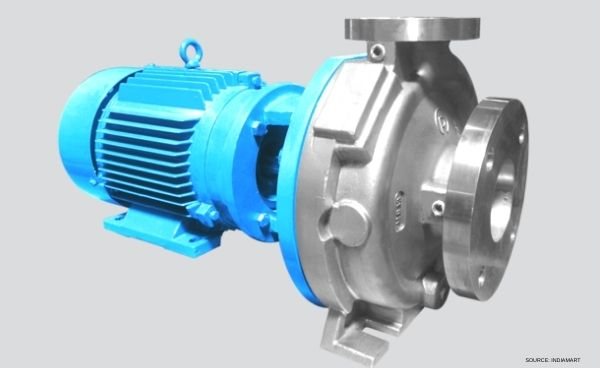# Important Pump Terminologypump is a device that moves fluids (liquids or gases), or sometimes slurries, by mechanical action, typically converted from electrical energy into Hydraulic energy.

Important Pumping terminology is described below.

#### 1) Head (h) [H]

Head is the expression of the energy content of a liquid in reference to an arbitrary datum. It is expressed in units of energy per unit weight of liquid. The measuring unit for the head is meters (feet) of liquid.

#### 2)Total head (H) [Htx]

This is the measure of energy increase, per unit weight of liquid, imparted to the liquid by the pump, and is the difference between total discharge head and total suction head.

This is the head normally specified for pumping applications because the complete characteristics of a system determine the total head required.

#### 3) Rate of flow [Q]

The rate of flow of a pump is the total volume throughput per unit of time at suction conditions. The term capacity is also used.

#### 4) Best Efficiency Point (BEP)

The rate of flow and total head at which the pump efficiency is maximum at a given speed and impeller diameter.

#### 5) Displacement (D)

For a positive displacement pump it is the theoretical volume per revolution of the pump shaft. Calculation methods and terminology may differ between different types of positive displacement pumps.

#### 6) Net Positive Suction Head Available (NPSHA)

NPSHA is determined by the conditions of the installation and is the total suction head of liquid absolute, determined at the first-stage impeller datum minus the absolute vapor pressure in meters (feet) of the liquid at a specific rate of flow expressed in meters (feet) of liquid.

Note that for positive displacement pumps the term Net Positive Inlet Pressure Available (NPIPA) is used and is expressed in pressure absolute kPa (psi).

#### 7) Net Positive Suction Head Required (NPSHR)

NPSHR is the minimum NPSH given by the manufacturer/supplier for a pump achieving a specified performance at the specified capacity, speed, and pumped liquid. Note that occurrence of visible cavitation, increase of noise and vibration due to cavitation, beginning of head or efficiency drop, and cavitation erosion can occur when margin above NPSHr is present.

Note that for positive displacement pumps the term Net Positive Inlet Pressure Required (NPIPR) is expressed in pressure absolute kPa (psi).

#### 8) Net Positive Suction Head 3% (NPSH3)

For rotodynamic pumps, NPSH3 is defined as the value of NPSHR at which the first-stage total head drops by 3% due to cavitation.

This is determined by the vendor by testing with water as outlined in. ANSI/HI 14.6 Rotodynamic Pumps for Hydraulic Performance Acceptance Tests

#### 9) Suction specific speed (S)

Suction specific speed is an index of pump suction operating characteristics. It is determined at the BEP rate of flow with the maximum diameter impeller. Suction specific speed is an indicator of the net positive suction head required [NPSH3] for given values of capacity and also provides an assessment of a pump’s susceptibility to internal recirculation. Suction specific speed is expressed by the following equation: Suction Specific Speed.